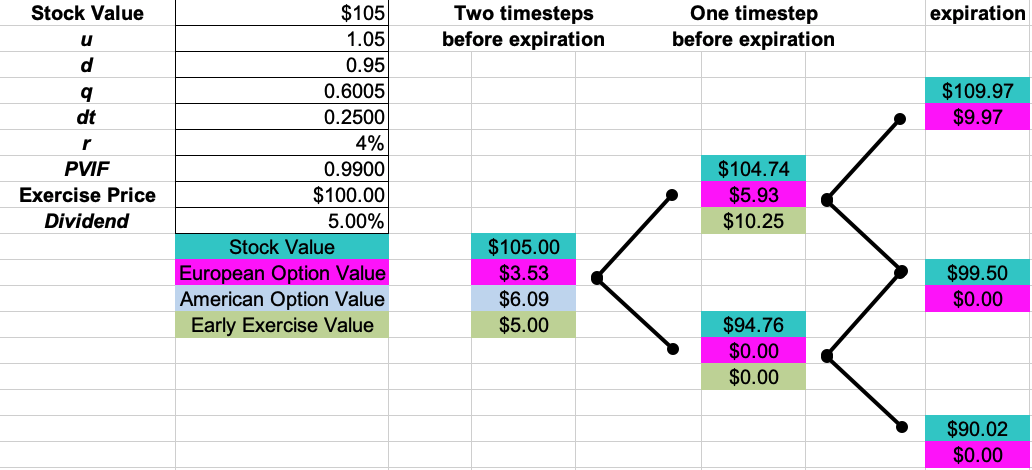# Today’s class problem: American Call Option on dividend paying Stock

Today’s class problem (see the last page) of the annotated “Finance 4366 – Early Exercise of American Call and Put options – no dividend” lecture note – there, we modified the two time-step call option pricing problem so that instead of having no dividend, we assumed that the underlying stock pays a 5% dividend of its price at either the or the node.  Without a dividend, it doesn’t pay to exercise an American call option early, so the value of an American call on a non-dividend paying stock is the same as the value of a European call on the said stock.

As we saw in today’s lecture, dividend payments have the effect of slowing down the rate of growth of the underlying asset.  As shown in the “Finance 4366 – Early Exercise of American Call and Put options – no dividend” lecture note, without a dividend, then the beginning share price of \$105 grows to \$110.25 at the u node and declines to \$99.75 at the d node.  Subsequently, the share price at the uu node grows to \$115.76, declines to \$104.74 at the ud node, and declines to \$94.76 at the dd node.  Applying risk neutral valuation, we find that the value of the American call is equal to the value of the European call is equal to \$7.80, because it is not worthwhile to exercise the American call at the beginning of the binomial tree or at nodes u or d.  However, the payment of a 5% dividend after one time-step changes everything, as shown by the following spreadsheet:Here, when a 5% dividend payment occurs after one time-step, then the ex-dividend price of the stock is \$104.75 at node u and \$94.76 at node d.  Subsequently, the share price at the uu node grows to only \$109.97 at node uu, declines to \$99.50 at the ud node, and declines to \$90.02 at the dd node.  At node u, if the investor exercises the call option at the cum dividend price of \$110.25, then the investor earns \$10.25.  Since the arbitrage-free price of the call option is only  \$5.93 at node u, then the investor exercises the option at this node.  However, it is not profitable to exercise early at node d or at the inception of the option, as shown in the above spreadsheet.  In this case, the arbitrage-free price of a European option on the dividend-paying stock is$c = .99[.6005(5.93) + .3995(0)] = \ 3.53$, whereas the arbitrage-free price of a European option on the dividend-paying stock is$C = .99[.6005(10.25) + .3995(0)] = \ 6.09.$